The topic is very popular among the aptitude tests & IQ tests world wide. It is basically the application of the fundamental arithmetic concepts like percentage, ratio, average etc. The mixtures under consideration will be homogeneous in nature.

TYPES OF MIXTURES:- A mixture is not necessarily made up of liquids or solids. There can be various types such as.

• A liquid and a solid
• Two liquids
• Two solids
• Profit percent on two products
• Boys & girls in a group
• A journey covered with different speeds
• and many moreNo no….don’t get it wrong.
Here, we are talking about one of the interesting topics in CAT-preparation (and all others MBA entrance tests also) i.e. Circular motion – a small but very important part of a broader topic: Time, Speed and Distance.
We are specifically focusing here on the meetings of the runners on a circular track (or any closed track for that matter). As we go on, I will post some questions and in the explanation I’ll discuss few important concepts that’ll remain with you at the end of this session.Similar triangles present one of the biggest tools students have to solve many geometry questions. And yet, whenever the opportunity arises, the students often fail to spot the similarity between two triangles. Sometime they spot the similarity but fail to apply the ratios correctly. Today we will start with some familiar cases in which we apply similarity of triangles and then move on to some tricky and difficult cases. We hope that after solving all these problems, you’ll not have any issue in spotting and solving similarity.

In practical scenario, we always prove that two triangles are similar by proving that their corresponding angles are equal. For example, look at the very simple figure below:If you can prove that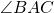=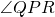and=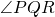then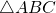and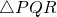are similar. In which case the ratios of the corresponding sides are equal. The best way to write the ratio of the sides is to write both the triangles in the order of the angle which are equal (for example here=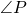,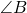=and=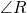so write ABC and PQR only) and then write the ratio by picking same corresponding points from the two written triangles. Therefore,Problems related to escalators baffle a lot of students preparing for CAT. Probably because not much time has been devoted to explaining this simple concept. Although we keep telling in our CAT classrooms continuously that escalator questions are similar to ‘upstream’ ‘downstream’ questions that students solve in time, speed and distance, the future MBAs still get confused because of the way that the questions are put. Today, we are going to see the theory behind escalator questions and solve them through both equations and ratios. In order to benefit the most, please solve these questions on your own before looking at the solutions.

An escalator is a moving stair, i.e. it moves continuously up or down. At any given point in time, the total number of stairs are fixed in an escalator. For example, for an escalator going up that is showing 50 steps, if 2 stairs disappear at the top, 2 stairs come out at the bottom. Most of the escalator questions involve people moving on an escalator. Remember that the speed of climbing stairs for a person does not vary whether the escalator is moving or still. For example, if Bantu can climb stairs at 2 stairs per second, his speed of climbing stairs on a moving escalator would stay the same.In CAT and other MBA entrance exams, there are many questions which fall in the domain of equations (quadratic, cubic, quartic..) and the properties of their roots. Deriving these properties will not only help us remember them but also give us the basic concepts to solve all kinds of equations and their problems. In this chapter we shall learn how the properties of roots of a general polynomial equation are derived. So let’s begin!

Let’s take a polynomial P(x) in a single variable x:

Letwhere,etc. are constants (associated with decreasing powers of x) and n, n-1, etc. are the whole number powers of the variable x. The highest power of the variable x is known as the ‘degree’ of the polynomial P(x).

For example,is a polynomial with degree 5 with